# Circular Reference issueHi All,

Please see attachment for details. My apologies my post encountered an error so I have to attach the details

Regards,

Tagged:

•It doesn't look like a composite hierarchy. So I would say lets create a subset of all Total/Average and ignore Parking portion from the list just to be on the safer side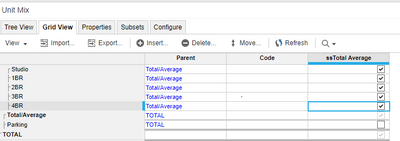Once you create a subset create below module. I created additional two line items so that they help me in getting me the correct output at top level by having RATIO at the summary level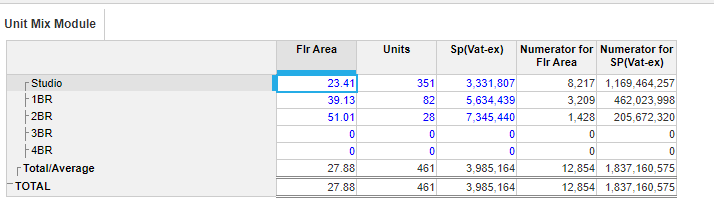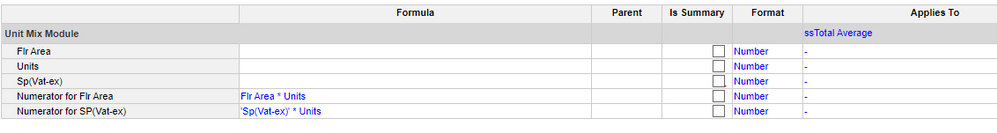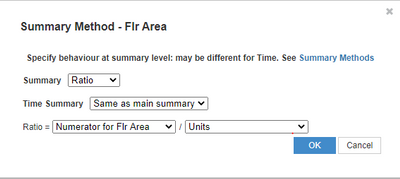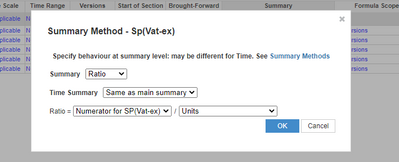Hope I got the requirement correct and hope that it helps

Misbah

•Hi @Jsdeloria21,

Can you clarify my doubts so that i will analyse your problem well.

1. i assume Flr Area, units, SP(VAT -ex) are line items and Unit Mix is a dimension of a module.

2. Total/Average (Flr Area) = (Flr Area (Studio)*Unit(Studio)) + (Flr Area (1 BR) *Unit (1BR))+ (Flr Area(2BR)*Unit(2BR)) + (Flr Area (3BR))*Unit(3BR)) + Flr Area(4BR) * Unit *4BR)) /Unit(Total/Average

is it a formula written on Flr Area line item...?

3. Total/Average (SP(VAT -ex))=(SP VAT-ex (Studio)*Unit(Studio)) + (SP VAT-ex (1 BR) *Unit (1BR))+ (SP VAT-ex(2BR)*Unit(2BR)) + (SP VAT-ex (3BR))*Unit(3BR)) + SP VAT-ex (4BR) * Unit *4BR)) /Unit(Total/Average

is it a formula written on SP(VAT -ex) line item...?

4. I tried to use Flr Area[select:Studio]*Units[select:Studio] and I got the Circular reference

in which module are you writing this formula..?

and what is the line item name...?

Thanks,